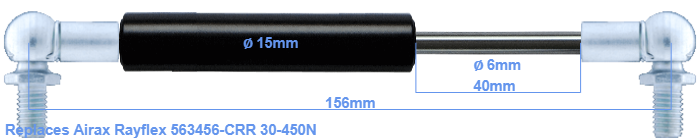# Replacement for Airax Rayflex 563456-CRR 30-450N

\$38.05

Replacement gas spring for the Airax Rayflex 563456-CRR 30-450 Newton. This fits a (possibly already present) ball with a diameter of 10mm. A corresponding ball is included. Brand: Stabilus Industry Line.
 Force Choose an option30 Newton40 Newton50 Newton60 Newton80 Newton100 Newton120 Newton140 Newton150 Newton160 Newton180 Newton200 Newton220 Newton240 Newton250 Newton260 Newton280 Newton300 Newton320 Newton340 Newton350 Newton360 Newton380 Newton400 Newton420 Newton440 Newton450 NewtonClear
This gas spring is also known as 5000563456-CRR, 563456-CRR.00.The diameter of the cylinder of this gas spring is 15 mm. The cross section of the rod is 6 mm. The stroke is 40 millimeter long. The total length of this replacement is exactly 156 mm. This is the distance between the centers of the mounting parts. Without the mounting parts this gas spring is 112 mm long (thread to thread). This replacement gas spring (563456-CRR) has a force of 30-450 Newton (N). Attention: this is not an official Airax Rayflex gas spring, but a replacement gas spring. This gas spring was produced by Stabilus subsidiary Hahn with the brand Stabilus Industry Line. The gas spring can be used without problems as a replacement, because the dimensions and force equal.
Category: